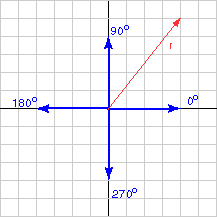• |r| = | (4,5)T | = (16+25) = 6.40
• direction = arc tan{ 5/4 } = 51.34°

# Length and Direction converted to (x, y)Say that you have been given a length and a direction and want to express them as a 2D column vector.

1. Draw a sketch.
2. Calculate x by projecting the length onto the x-axis
(usually length*cos( θ ) )
3. Calculate y by projecting the length onto the y-axis
(usually length*sin( θ ) )
4. Check answers against the sketch.

Of course, you can't wait to practice this (it brings back those fond memories of trigonometry.)

### QUESTION 7:

A vector has length 4 and orientation 150°. Represent the vector as a column matrix.